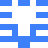### Real Vim ninjas count every keystroke - do you?

###### Pick a challenge, fire up Vim, and show us what you got.

```Your VimGolf key: please sign in

\$ gem install vimgolf
\$ vimgolf setup
\$ vimgolf put 9v006233d72d000000000219
```

### Fix the shell script!

Fix the shell script with the fewest keystrokes!

##### Start file
```#!/bin/bash
a = 5
b = 10
sum = \$a + \$b
echo \$sum

mul = \$a * \$b
echo \$mul
```
##### End file
```#!/bin/bash
a=5
b=10
sum=\$((a + b))
echo \$sum

mul=\$((a * b))
echo \$mul
```

#### View Diff

```2,4c2,4
< a = 5
< b = 10
< sum = \$a + \$b
---
> a=5
> b=10
> sum=\$((a + b))
7c7
< mul = \$a * \$b
---
> mul=\$((a * b))
```

### Solutions by @tmdals5512:

Unlock 1 remaining solutions by signing in and submitting your own entry

## 450 active golfers, 1852 entries

##### Solutions by @tmdals5512:28
###### #109 - 박승민 / @tmdals5512

04/17/2022 at 08:50AM